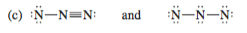# Problem: In each of the following pairs, determine whether the two represent resonance contributors of a single species or depict different substances. If two structures are not resonance contributors, explain why.

###### Problem Details

In each of the following pairs, determine whether the two represent resonance contributors of a single species or depict different substances. If two structures are not resonance contributors, explain why.What scientific concept do you need to know in order to solve this problem?

Our tutors have indicated that to solve this problem you will need to apply the Resonance Structures-1 concept. If you need more Resonance Structures-1 practice, you can also practice Resonance Structures-1 practice problems.

What is the difficulty of this problem?

Our tutors rated the difficulty ofIn each of the following pairs, determine whether the two re...as low difficulty.

How long does this problem take to solve?

Our expert Organic tutor, Jonathan took 1 minute and 15 seconds to solve this problem. You can follow their steps in the video explanation above.

What textbook is this problem found in?

Our data indicates that this problem or a close variation was asked in Organic Chemistry - Carey 8th Edition. You can also practice Organic Chemistry - Carey 8th Edition practice problems.